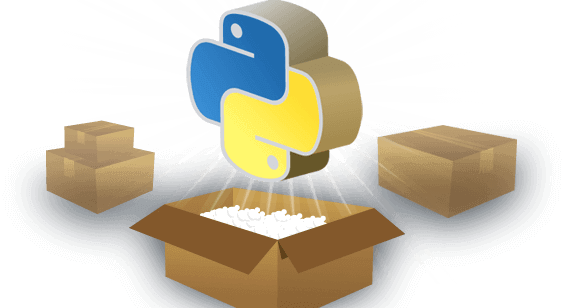# Python基础07 循环

python编程中，Python提供了for循环和while循环，本篇python基础教程介绍python循环语句，

在程序开发中，程序的运行流程共有三种方式：顺序 — 从上向下，顺序执行代码；分支 — 根据条件判断，决定执行代码的分支；循环 — 让特定代码重复执行，python循环语句允许我们执行一个语句或语句组多次或者更复杂的执行路径。

python循环控制流程图：

#### python for循环

Python3中for循环语句的一般形式如下所示：

``````#!/usr/bin/python3

for keyword in keywords:
break
print("循环数据 " + keyword)
else:
print("没有循环数据!")
print("完成循环!")

``````

for 实例中使用了 break 语句，break 语句用于跳出当前循环体

for 循环打印乘法口诀:

``````#!/usr/bin/python3

for i in range (1,10):
for j in range (1,i+1):
print("{}*{}={}".format(j,i,i*j),end='\t')
print()

1*1=1
1*2=2	2*2=4
1*3=3	2*3=6	3*3=9
1*4=4	2*4=8	3*4=12	4*4=16
1*5=5	2*5=10	3*5=15	4*5=20	5*5=25
1*6=6	2*6=12	3*6=18	4*6=24	5*6=30	6*6=36
1*7=7	2*7=14	3*7=21	4*7=28	5*7=35	6*7=42	7*7=49
1*8=8	2*8=16	3*8=24	4*8=32	5*8=40	6*8=48	7*8=56	8*8=64
1*9=9	2*9=18	3*9=27	4*9=36	5*9=45	6*9=54	7*9=63	8*9=72	9*9=81
``````

#### python while循环

Python3中 while 循环语句的一般形式如下所示：

``````#!/usr/bin/python3

i = 1

while i < 5:
print("这是最好的时代")
i= i+1
print("循环结束，再来一次")

``````

break 语句可以跳出 for 和 while 的循环体。如果你从 for 或 while 循环中终止，任何对应的循环 else 块将不执行。在while 循环中，如果条件一直为真的话，程序会一直运行下去，相当于无限循环，这个没必要的，所以一般要加个break语句来终止程序。

while 循环无限循环示例：

``````#!/usr/bin/python3

flag = 1

while flag:
print("这是最好的时代")

......
``````

continue 语句被用来告诉 Python 跳过当前循环块中的剩余语句，然后继续进行下一轮循环。

推荐阅读：零基础如何开始学习Python